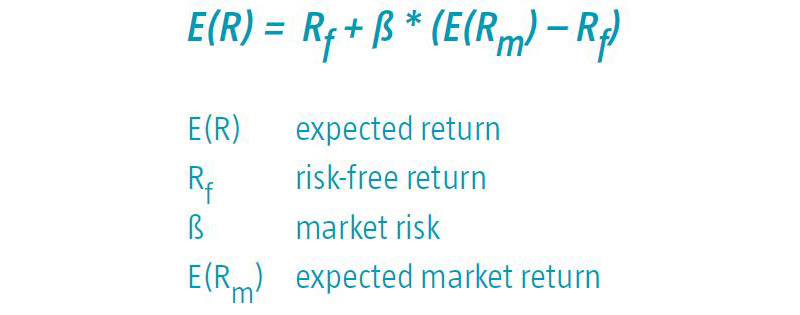Quantitative investing

# Capital Asset Pricing Model

The Capital Asset Pricing Model (CAPM) is the product of a financial investment theory that reflects the relationship between risk and expected return. The model assumes a linear relationship.

### The capital asset pricing model formula for calculating expected return is:The Capital Asset Pricing Model is used to forecast returns that can be obtained with risk-bearing asset classes. The linear relationship means that taking extra risk will on average lead to higher returns.

However, empirical tests performed in the early seventies* with this capital asset pricing model showed that the relationship between risk and return is less strong than the theory indicates.

* One of the first tests was a study performed by Haugen and Heins: ‘On the Evidence Supporting the Existence of Risk Premiums in the Capital Market’ (1972). They demonstrated that over the period 1929 - 1971, low-volatility equities realized extra risk-adjusted returns.